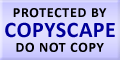Submit Assignment From Here
Name
:
Email
:
Phone
:
Country
:
Willing to pay US\$
:
Subject
:
Topic
:
Levels
:
:Hours
:

# Sampling Distributions and Estimation Homework Help

While performing an experiment the outcome of the numerical variable is random. Now if we repeat the above performed experiment we will get a new random variable, but this variable will be independent of the previous variable. Hence from the above analysis we can say that the above generated new random variables are “independent and identically distributed”. In short we can say that the above two variable are ‘idd’. The performance of the experiment gives us the distribution, but every new trial of same experiment provides us with a new variable. So, we can conclude that if we perform this activity n no. of time we will get n “independent and identically distributed’ variables.
For example, we can consider a coin tossing experiment where the result will be random without any certain outcomes, the coin has head = p which is uncertain. Symbolically we can represent it as:
A rv X is

We need this equation because an rv must be in numerical form. Now, if we repeat this experiment 4 more times the result will be 4 different random variable. X1, X2, … , X4 all these variable are independent and have the same distribution of coin tossing. We can conclude that a statistics is a random variable and has a distribution and this distribution of statistics is known as sampling distribution.Make Payment OnlineUS